Constructing a triangle using RHS congruency criteria

Practical Geometry
Serial order wise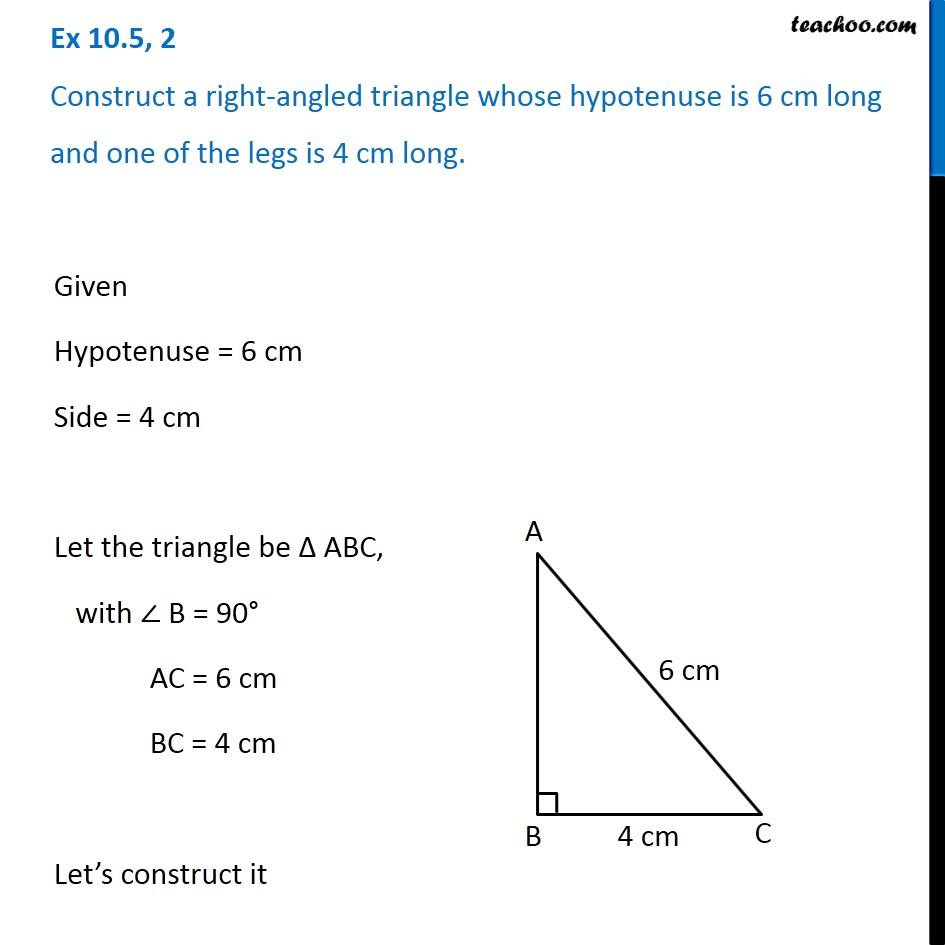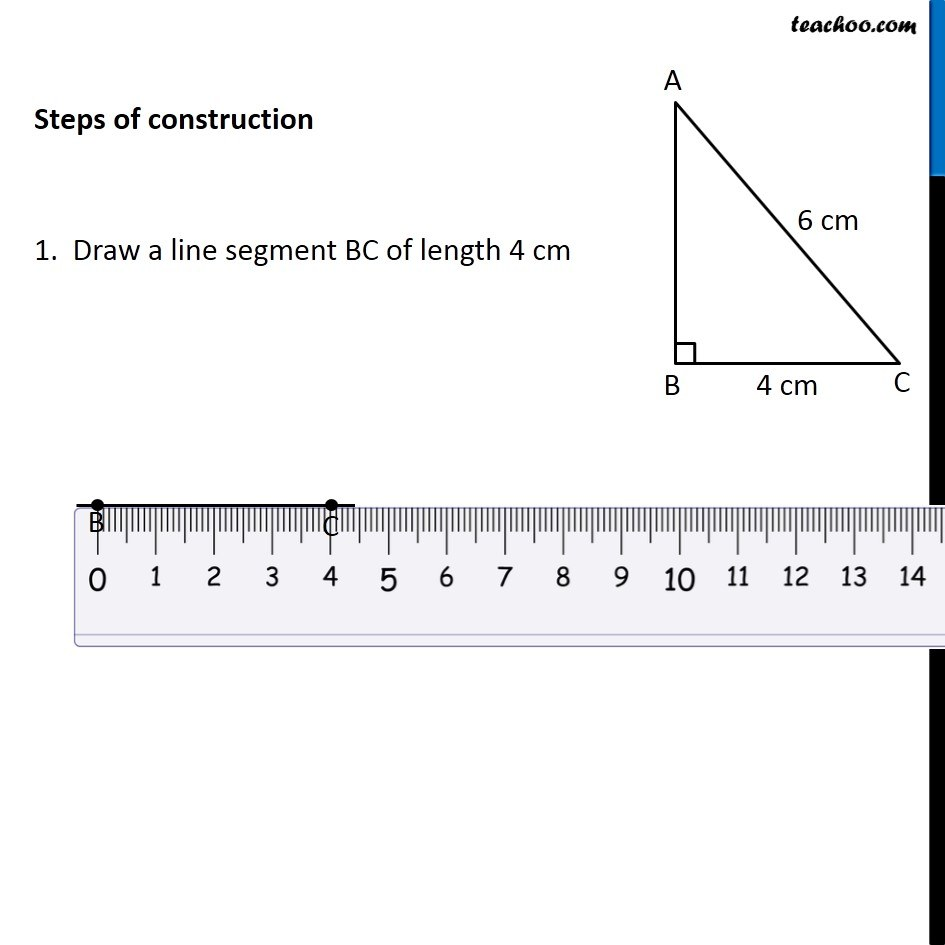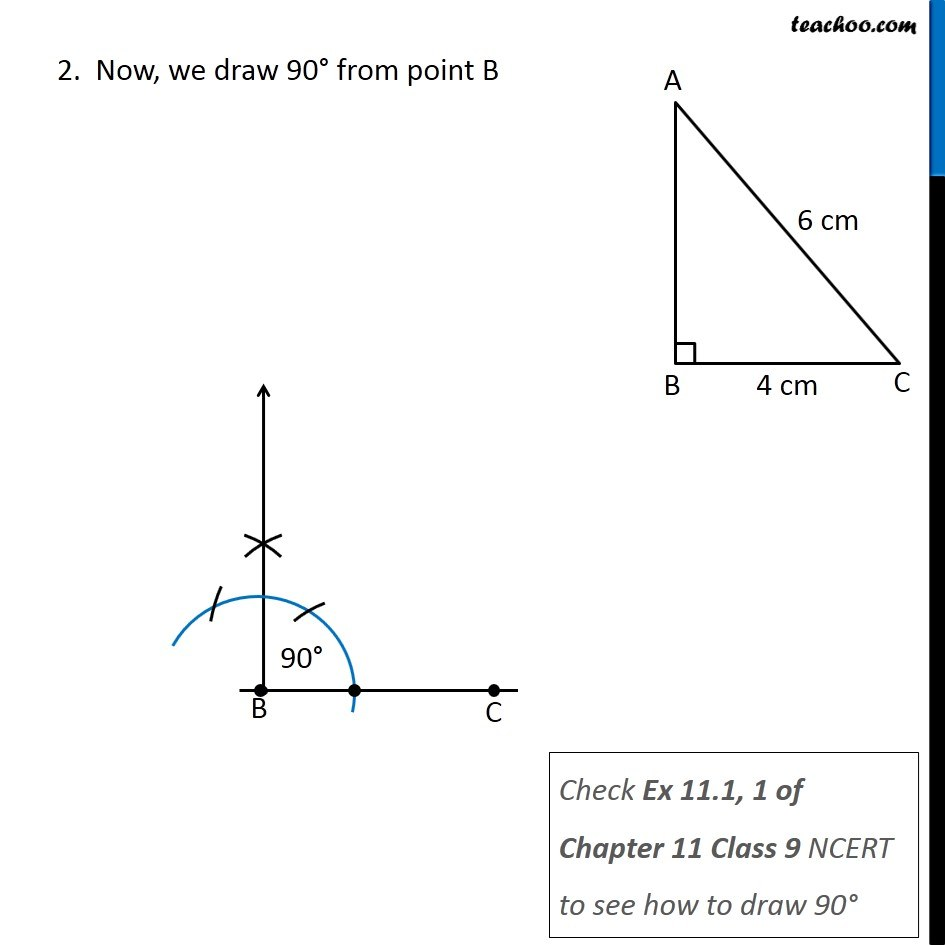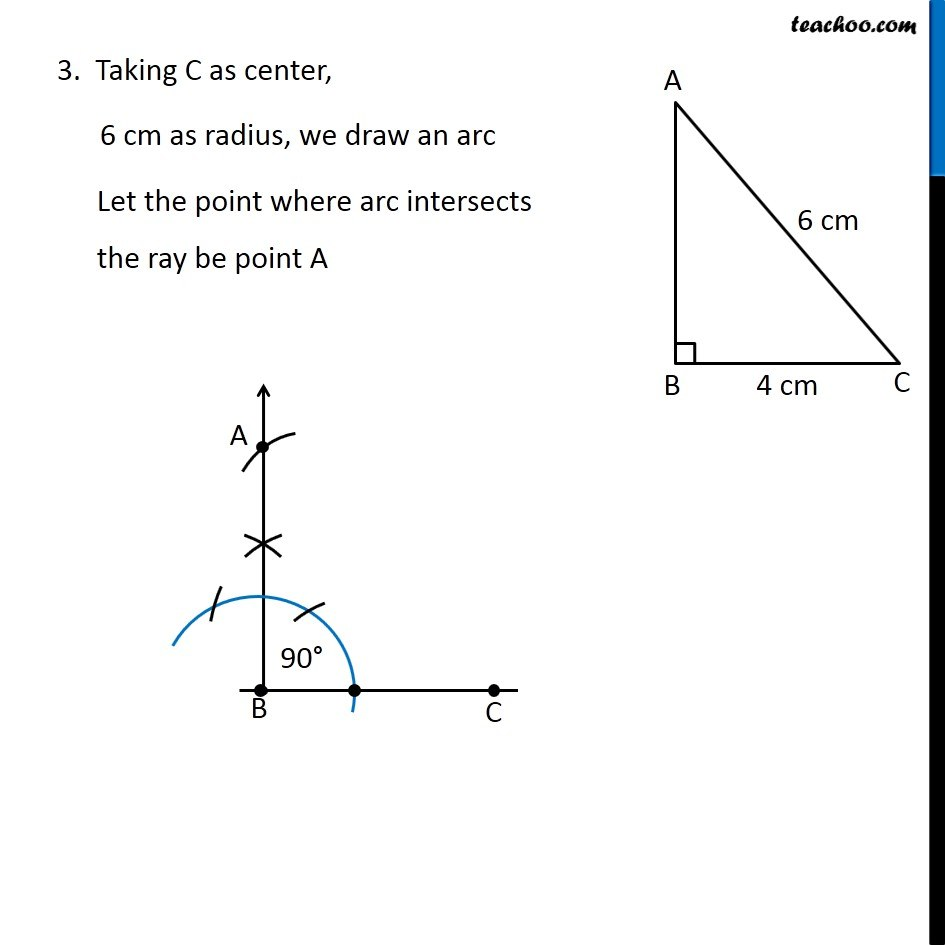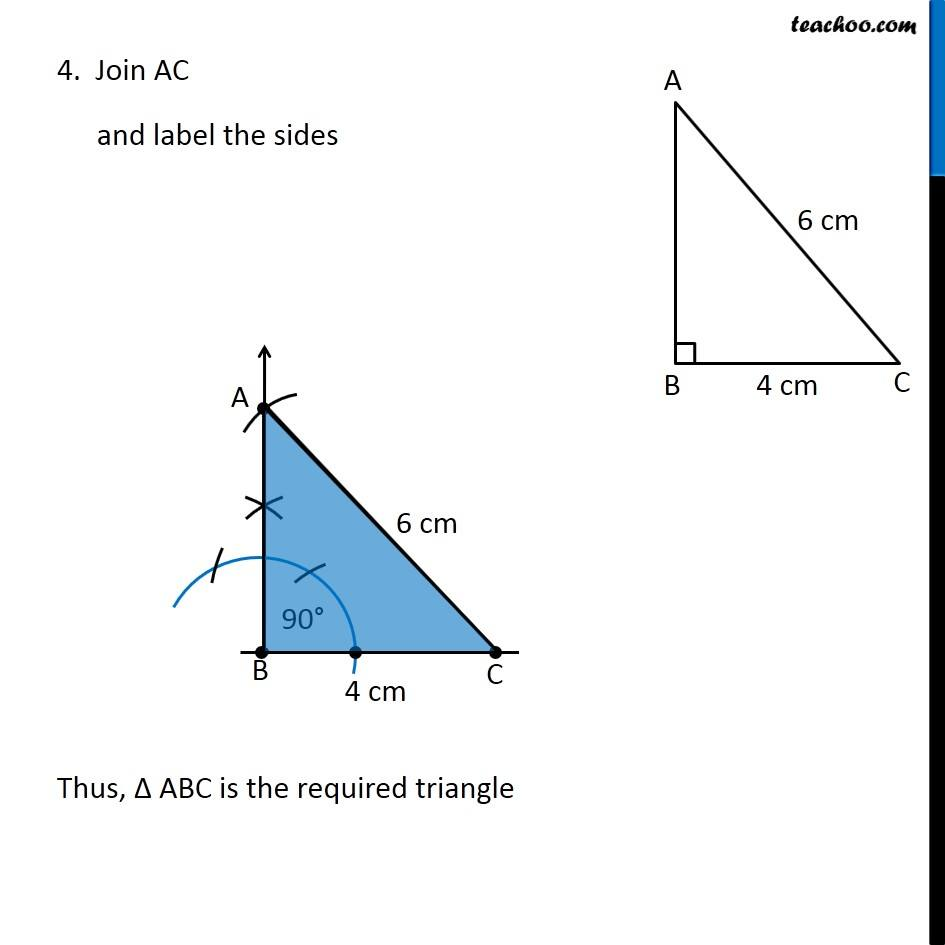Learn in your speed, with individual attention - Teachoo Maths 1-on-1 Class

### Transcript

Question 2 Construct a right-angled triangle whose hypotenuse is 6 cm long and one of the legs is 4 cm long. Given Hypotenuse = 6 cm Side = 4 cm Let the triangle be Δ ABC, with ∠ B = 90° AC = 6 cm BC = 4 cm Let’s construct it Steps of construction 1. Draw a line segment BC of length 4 cm 2. Now, we draw 90° from point B Check Ex 11.1, 1 of Chapter 11 Class 9 NCERT to see how to draw 90° 3. Taking C as center, 6 cm as radius, we draw an arc Let the point where arc intersects the ray be point A 4. Join AC and label the sides Thus, Δ ABC is the required triangle